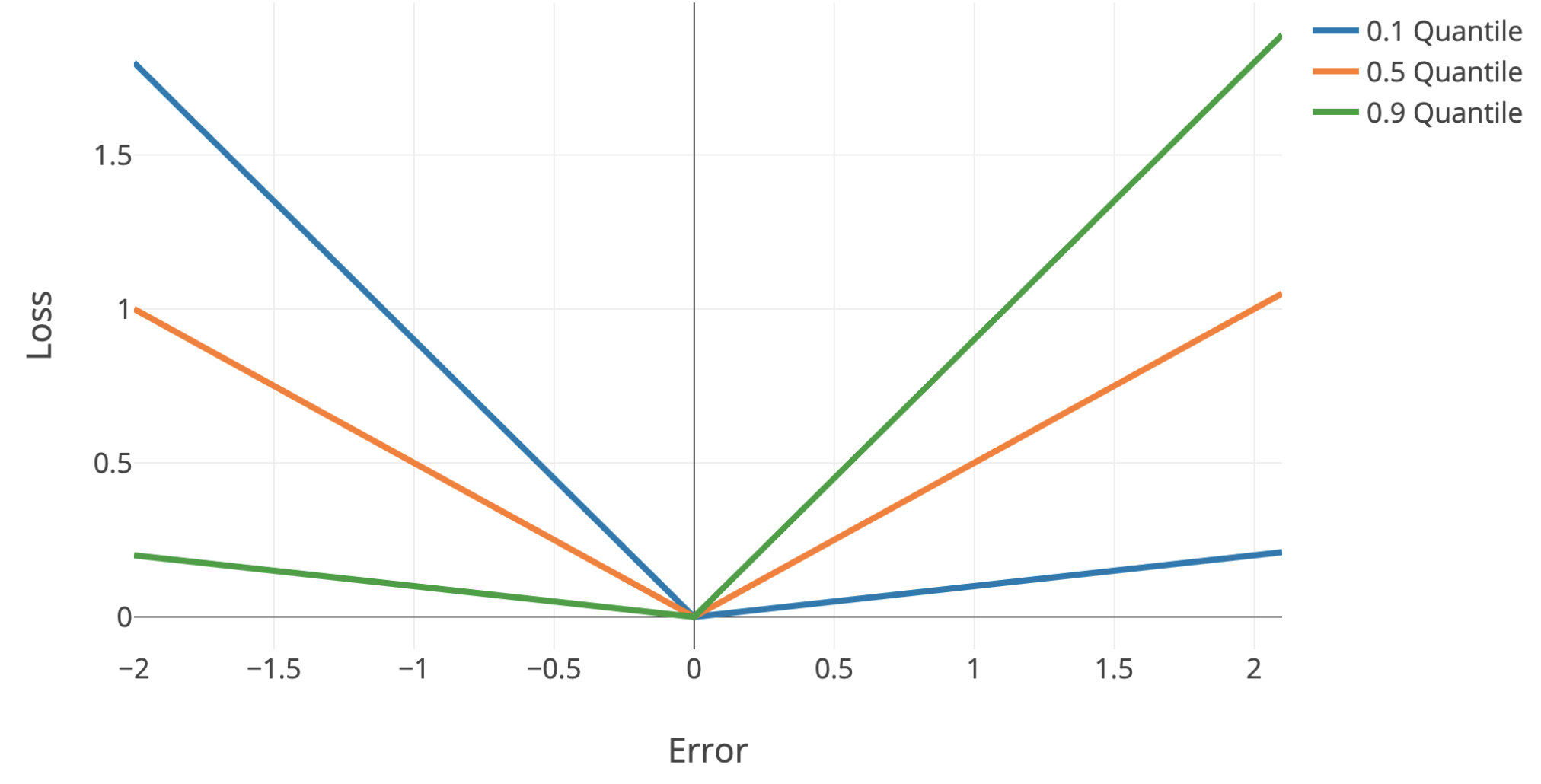uncertainty

some notes on uncertainty in machine learning, particularly deep learning

# basics

• calibration - predicted probabilities should match real probabilities
• platt scaling - given trained classifier and new calibration dataset, basically just fit a logistic regression from the classifier predictions -> labels
• isotonic regression - nonparametric, requires more data than platt scaling
• piecewise-constant non-decreasing function instead of logistic regression
• confidence - predicted probability = confidence, max margin, entropy of predicted probabilities across classes
• ensemble uncertainty - ensemble predictions yield uncertainty (e.g. variance within ensemble)
• quantile regression - use quantile loss to penalize models differently + get confidence intervals
• can easily do this with sklearn
• quantile loss = $\begin{cases} \alpha \cdot \Delta & \text{if} \quad \Delta > 0\\(\alpha - 1) \cdot \Delta & \text{if} \quad \Delta < 0\end{cases}$
• $\Delta =$ actual - predicted
•• Single-Model Uncertainties for Deep Learning (tagovska & lopez-paz 2019) - use simultaneous quantile regression

# outlier-detection

Note: outlier detection uses information only about X to find points “far away” from the main distribution

• overview from sklearn
• elliptic envelope - assume data is Gaussian and fit elliptic envelop (maybe robustly) to tell when data is an outlier
• local outlier factor (breunig et al. 2000) - score based on nearest neighbor density
• idea: gradients should be larger if you are on the image manifold
• isolation forest (liu et al. 2008) - lower average number of random splits required to isolate a sample means more outlier
• one-class svm - estimates the support of a high-dimensional distribution using a kernel (2 approaches:)
• separate the data from the origin (with max margin between origin and points) (scholkopf et al. 2000)
• find a sphere boundary around a dataset with the volume of the sphere minimized (tax & duin 2004)
• detachment index (kuenzel 2019) - based on random forest
• for covariate $j$, detachment index $d^j(x) = \sum_i^n w (x, X_i) \vert X_i^j - x^j \vert$
• $w(x, X_i) = \underbrace{1 / T\sum_{t=1}^{T}}{\text{average over T trees}} \frac{\overbrace{1{ X_i \in L_t(x) }}^{\text{is } X_i \text{ in the same leaf?}}}{\underbrace{\vert L_t(x) \vert}{\text{num points in leaf}}}$ is $X_i$ relevant to the point $x$?

# uncertainty detection

Note: uncertainty detection uses information about X / $\phi(X)$ and Y, to find points for which a particular prediction may be uncertain. This is similar to the predicted probability output by many popular classifiers, such as logistic regression.

• rejection learning - allow models to reject (not make a prediction) when they are not confidently accurate (chow 1957, cortes et al. 2016)
• To Trust Or Not To Trust A Classifier (jiang, kim et al 2018) - find a trusted region of points based on nearest neighbor density (in some embedding space)
• trust score uses density over some set of nearest neighbors
• do clustering for each class - trust score = distance to once class’s cluster vs the other classes

## bayesian approaches

• epistemic uncertainty - uncertainty in the DNN model parameters
• without good estimates of this, often get aleatoric uncertainty wrong (since $p(y\vert x) = \int p(y \vert x, \theta) p(\theta \vert data) d\theta$
• aleatoric uncertainty - inherent and irreducible data noise (e.g. features contradict each other)
• this can usually be gotten by predicting a distr. $p(y \vert x)$ instead of a point estimate
• ex. logistic reg. already does this
• ex. regression - just predict mean and variance of Gaussian
• gaussian processes

# conformal inference

• conformal inference constructs valid (wrt coverage error) prediction bands for individual forecasts
• relies on few parametric assumptions
• holds in finite samples for any distribution of (X, Y) and any algorithm $\hat f$
• starts with vovk et al. ‘90
• simple example: construct a 95% interval for a new sample (not mean) by just looking at percentiles of the empirical data
• empirical data tends to undercover (since empirical residuals tend to underestimate variance) - conformal inference aims to rectify this
• Uncertainty Sets for Image Classifiers using Conformal Prediction (angelopoulos, bates, malik, jordan, 2021)

# large language models (llms)

• Teaching Models to Express Their Uncertainty in Words (Lin et al., 2022) - GPT3 can generate both an answer and a level of confidence (e.g. “90% confidence”)
• Can LLMs Express Their Uncertainty? An Empirical Evaluation of Confidence Elicitation in LLMs (xiong et al. 2023)